# Twisty Puzzles

Twisty puzzles are puzzles that are inspired by Rubik's Cube.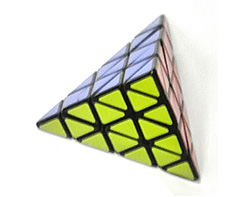Pyraminx 4x4 Tetrahedron Twisty Puzzle \$19.95 This "4 x 4" twisty puzzle is shaped like a regular tetrahedron, or triangular pyramid.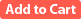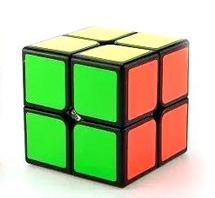2x2 Cube Twisty Puzzle \$7.95 A smooth-turning 2x2 version of Rubik's cube. A good starting place for exploring the world of twisty puzzles, this simple cube is not as easy as it looks.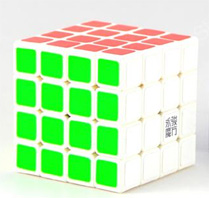4x4 Cube Twisty Puzzle \$14.95 A 4x4 version of Rubik's cube, with a white plastic body (MoYu brand).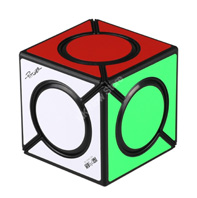Color Spots Twisty Puzzle \$9.95 The corner units turn on this unusual variation on Rubik's-type puzzles.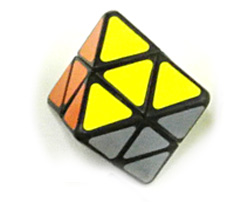Octahedron "2 x 2" Twisty Puzzle \$9.95 This "2 x 2" twisty puzzle is in the form of a regular octahedron (eight faces, each of which is an equilateral triangle).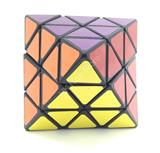Octahedron Twisty Puzzle \$14.95 This twisty puzzle is in the form of a regular octahedron (eight faces, each of which is an equilateral triangle).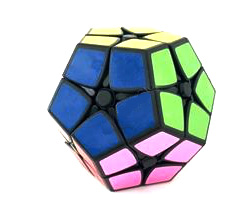Dodecahedron "2 x 2" Twisty Puzzle \$12.95 This "2 x 2" twisty puzzle is in the form of a regular dodecahedron.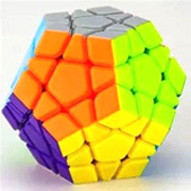Megaminx Dodecahedron Puzzle \$19.95 The 12 different colors of this high-quality rotational puzzle should throw you for a loop as to the solution. This version has 12 different colors of plastic rather than stickers. MoYu brand, 3 1/4 in x 3 1/4 in x 2 2/3 in.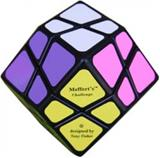Rhombic Dodecahedron Twisty Puzzle \$14.95 This twisty puzzle is in the form of a rhombic dodecahedron (twelve faces, each of which is a rhombus).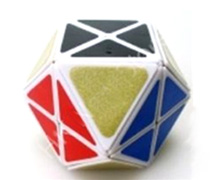Cuboctahedron Twisty Puzzle \$14.95 This "face-turning" twisty puzzle is in the form of a cuboctahedron (eight triangular faces and six square faces).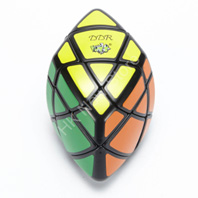Pillow Rhombohedron Twisty Puzzle \$19.95 This puzzle is in the form of a rhombohedron (six faces, each of which is a rhombus), with the faces bulged outward (are pillowed).